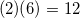# High School Math : How to find the endpoints of a line segment

## Example Questions

### Example Question #15 : Midpoint And Distance Formulas

What is the length of a line with endpoints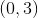and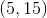?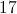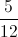Explanation:

The formula for the length of a line is very similiar to the pythagorean theorem: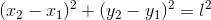Plug in our given numbers to solve: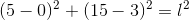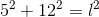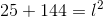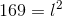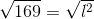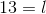### Example Question #16 : Midpoint And Distance Formulas

The points A, B, and C reside on a line segment. B is the midpoint of AC. If line AB measures 6 units in length, what is the length of line AC?Explanation:

If B is the midpoint of AC, then AC is twice as long as AB. We are told that AB=6.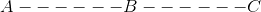The diagram shows six units between points A and B, with B as the midpoint of segment AC. Therefore segment BC is also six units long, so line AC is twelve units long.